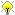I’ve been putting off revising the Rings & Arithmetic course since I didn’t follow the lectures or really do the problems back in Michaelmas, but people keep telling me it’s nice and actually quite easy, so I’m finally doing some—it’s a compulsory course, but I only have to answer one question so I thought I might just skip it and try and put more time into the others. I think that having done some Number Theory helps a great deal. Fellow Hungarian mathematician who did most of the second year number theory course at schoolsays this helped a lot; they did this while we were wasting our time with calculus.

So I’m starting to like algebra more—especially now that quotient spaces don’t scare me quite so much—but this is the usual thing that I really like Maths when I can do it, but I can’t do it often enough in this degree course that I stop enjoying it. I wish I’d had a better year so I could have a better grasp of courses like this; I should have persevered with Fields, too. Also for any maths undergrads reading, Abstract Algebra by I.N. Herstein (Chichester: Wiley 1999) is definitely worth checking out; doesn’t quite do enough for my second year rings course, but shows you what’s important about the topics.

Here’s the coolest thing I’ve found so far, where we use quotients of rings to construct ℂ, rather than just saying that there’s an element i that squares to  − 1, which appeals to my constructivist leanings.

We have the ring of polynomials with real coefficients, ℝ[X], no problem, and now write ⟨X2 + 1⟩ for the multiples of the polynomial X2 + 1. It’s easy to show that $\langle X2+1\rangle \vartriangleleft \mathbb{R}[X]$. So we can form the quotient ring ℝ[X]/⟨X2 + 1⟩, and this is essentially ℂ. So what’s our imaginary unit? Well write $\mathrm{i} \mathrel{\mathop:}= \overline{X} \mathrel{\mathop:}= X + \langle X2+1\rangle$ and then using the division algorithm we can get that every element can be written a + b**i: every polynomial may be written as a + b**X + g(X)(X2 + 1)). And indeed

$$\mathrm{i}^2 = \overline{X}^2 = \overline{X2} = \overline{X2 + 1 - 1} = \overline{X2 + 1} - \overline{1} = 0 - 1 = -1$$

as we require.

 The reason we use X rather than x here is to emphasise that polynomials are not functions, just formal expressions of coefficients and a few X. x would traditionally denote a variable in a function rather than just a component of an expression.

comment NVWB9OIOQGDX7ZUN

It’s strange: for me, this sort of construction of i is just the same, really as the “standard” “this is a number which squares to -1” non-construction. Feels just as arbitrary or not.

Comment by Thu 16 Jun 2011 01:56:45 UTC